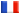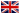# Séminaire de Cryptographie

## Maike Massierer### Is there an L(1/4) algorithm for the discrete logarithm problem in algebraic curves?

The function field sieve, an algorithm of subexponential complexity L(1/3) that computes discrete logarithms in finite fields, has recently been improved to an L(1/4) algorithm, and subsequently to a quasi-polynomial time algorithm. Since index calculus algorithms for computing discrete logarithms in Jacobians of algebraic curves are based on very similar concepts and results, the natural question arises whether the recent improvements of the function field sieve can be applied in the context of algebraic curves. While we are not able to give a final answer to this question at this point, since this is work in progress, we discuss a number of ideas, experiments, and possible conclusions.